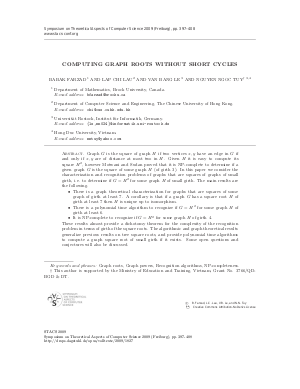Document# Computing Graph Roots Without Short Cycles

### Authors Babak Farzad, Lap Chi Lau, Van Bang Le, Nguyen Ngoc Tuy## File

LIPIcs.STACS.2009.1827.pdf
• Filesize: 181 kB
• 12 pages

## Cite As

Babak Farzad, Lap Chi Lau, Van Bang Le, and Nguyen Ngoc Tuy. Computing Graph Roots Without Short Cycles. In 26th International Symposium on Theoretical Aspects of Computer Science. Leibniz International Proceedings in Informatics (LIPIcs), Volume 3, pp. 397-408, Schloss Dagstuhl - Leibniz-Zentrum für Informatik (2009)
https://doi.org/10.4230/LIPIcs.STACS.2009.1827

## Abstract

Graph $G$ is the square of graph $H$ if two vertices $x,y$ have an edge in $G$ if and only if $x,y$ are of distance at most two in $H$. Given $H$ it is easy to compute its square $H^2$, however Motwani and Sudan proved that it is NP-complete to determine if a given graph $G$ is the square of some graph $H$ (of girth $3$). In this paper we consider the characterization and recognition problems of graphs that are squares of graphs of small girth, i.e. to determine if $G=H^2$ for some graph $H$ of small girth. The main results are the following. \begin{itemize} \item There is a graph theoretical characterization for graphs that are squares of some graph of girth at least $7$. A corollary is that if a graph $G$ has a square root $H$ of girth at least $7$ then $H$ is unique up to isomorphism. \item There is a polynomial time algorithm to recognize if $G=H^2$ for some graph $H$ of girth at least $6$. \item It is NP-complete to recognize if $G=H^2$ for some graph $H$ of girth $4$. \end{itemize} These results almost provide a dichotomy theorem for the complexity of the recognition problem in terms of girth of the square roots. The algorithmic and graph theoretical results generalize previous results on tree square roots, and provide polynomial time algorithms to compute a graph square root of small girth if it exists. Some open questions and conjectures will also be discussed.
##### Keywords
• Graph roots
• Graph powers
• Recognition algorithms
• NP-completeness

## Metrics

• Access Statistics
• Total Accesses (updated on a weekly basis)
0